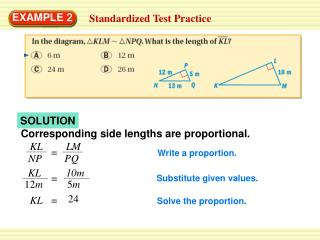# SOLUTION - PowerPoint PPT PresentationDownload PresentationSOLUTION

SOLUTIONDownload Presentation## SOLUTION

- - - - - - - - - - - - - - - - - - - - - - - - - - - E N D - - - - - - - - - - - - - - - - - - - - - - - - - - -
##### Presentation Transcript

1. KL LM = NP PQ KL 10m = 12m 5m 24 KL = EXAMPLE 2 Standardized Test Practice SOLUTION Corresponding side lengths are proportional. Write a proportion. Substitute given values. Solve the proportion.

2. EXAMPLE 2 Standardized Test Practice ANSWER The length of KL is 24 meters. The correct answer is C.

3. Alma is 5 feet tall and casts a 7 foot shadow. At the same time,a tree casts a 14 foot shadow. The triangles formed are similar. Find the height of the tree. EXAMPLE 3 Using Indirect Measurement Height

4. Tree’s height Length of tree’s shadow = Alma’s height Length of Alma’s shadow x feet 14feet = 5 feet 7 feet x 10 = ANSWER The tree is 10 feet tall. EXAMPLE 3 Using Indirect Measurement SOLUTION You can use a proportion to find the height of the tree. Write a proportion. Substitute given values. Solve the proportion.

5. Image Original (3x, 3y) (x, y) A(–1, –1) A’(– 3, – 3) B(0, 1) B’(0, 3) C’(6, 6) C(2, 2) D(3, 0) D’(9, 0) EXAMPLE 4 Dilating a Polygon Quadrilateral ABCD has vertices A(– 1, – 1), B(0, 1),C (2, 2), and D(3, 0). Dilate using a scale factor of 3. SOLUTION Graph the quadrilateral. Find the coordinates of the vertices of the image. Graph the image of the quadrilateral.

6. 2.At the right,ABCD , FGHJ. Find the value of x. ANSWER 8 x = for Examples 2, 3 and 4 GUIDED PRACTICE

7. What If? Suppose the tree in Example 3 casts a shadow of 18feet, a 6foot tall person casts a 9foot shadow, and the triangles are similar. Find the height of the tree. 3. ANSWER The tree is 12 feet tall. for Examples 2, 3 and 4 GUIDED PRACTICE

8. Graph RSTwith vertices R(1, 1),S(3, 2), and T(2, 3). Then graph its image after a dilation using a scale factor of 2. 4. ANSWER for Examples 2, 3 and 4 GUIDED PRACTICE